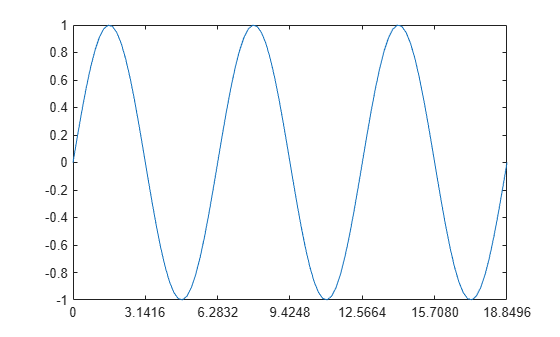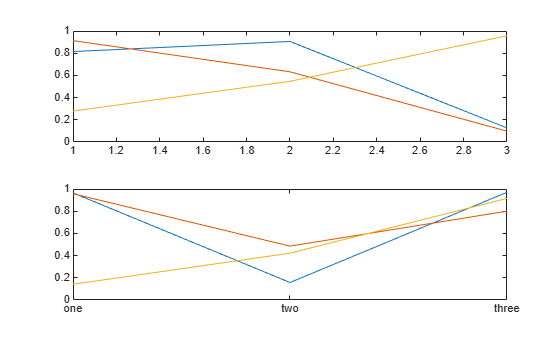# xticklabels

Set or query x-axis tick labels

## Syntax

xticklabels(labels)
xl = xticklabels
xticklabels('auto')
xticklabels('manual')
m = xticklabels('mode')
___ = xticklabels(ax,___)

## Description

example

xticklabels(labels) sets the x-axis tick labels for the current axes. Specify labels as a string array or a cell array of character vectors; for example, {'January','February','March'}. If you specify the labels, then the x-axis tick values and tick labels no longer update automatically based on changes to the axes.
xl = xticklabels returns the x-axis tick labels for the current axes.

example

xticklabels('auto') sets an automatic mode, enabling the axes to determine the x-axis tick labels. Use this option if you set the labels and then want to set them back to the default values.
xticklabels('manual') sets a manual mode, freezing the x-axis tick labels at the current values.
m = xticklabels('mode') returns the current value of the x-axis tick labels mode, which is either 'auto' or 'manual'. By default, the mode is automatic unless you specify the tick labels or set the mode to manual.

example

___ = xticklabels(ax,___) uses the axes specified by ax instead of the current axes. Specify ax as the first input argument for any of the previous syntaxes.

## Examples

collapse all

Create a line plot. Display tick marks along the x-axis at the values 0, 5, and 10. Then specify a label for each tick mark.

x = linspace(0,10); y = x.^2; plot(x,y) xticks([0 5 10]) xticklabels({'x = 0','x = 5','x = 10'})Create a line plot. Specify the x-axis limits and display tick marks along at the x-axis at increments of $\pi$.

x = linspace(0,6*pi); y = sin(x); plot(x,y) xlim([0 6*pi]) xticks(0:pi:6*pi)MATLAB® labels the tick marks with the numeric values. Change the labels to show the $\pi$ symbol by specifying text for each label.

xticklabels({'0','\pi','2\pi','3\pi','4\pi','5\pi','6\pi'})Starting in R2019b, you can display a tiling of plots using the tiledlayout and nexttile functions. Call the tiledlayout function to create a 2-by-1 tiled chart layout. Call the nexttile function to create the axes objects ax1 and ax2. Create two plots of random data. Set the x-axis tick values and labels for the second plot by specifying ax2 as the first input argument.

tiledlayout(2,1) ax1 = nexttile; plot(ax1,rand(3)) ax2 = nexttile; plot(ax2,rand(3)) xticks(ax2,[1 2 3]) xticklabels(ax2,{'one','two','three'})Create a stem chart, and specify the x-axis tick values and corresponding labels. Then set the x-axis tick values and labels back to the default values.

stem(1:10) xticks([1 4 6 10]) xticklabels({'A','B','C','D'})xticks('auto') xticklabels('auto')Remove the tick labels along the x-axis by specifying the tick labels as an empty array.

plot(rand(5)) xticklabels({})## Input Arguments

collapse all

Tick labels, specified as a cell array of character vectors, string array, or categorical array. If you do not want tick labels to show, then specify an empty cell array {}. Tick labels support TeX and LaTeX markup. See the TickLabelInterpreter property of the Axes object for more information.

Example: xticklabels({'0','\pi','2\pi'})

Example: xticklabels({'January','Febrary','March'})

Example: xticklabels({})

Note

• To specify the tick values, use the xticks function.

• If you do not specify enough labels for all the ticks values, MATLAB® uses the labels followed by empty character vectors for the remaining ticks.

• If you specify the tick labels as a categorical array, MATLAB uses the values in the array, not the categories.

Target axes, specified as an Axes object or an array of Axes objects.

If you do not specify this argument, then xticklabels modifies the current axes.

## Output Arguments

collapse all

Current tick labels, returned as a cell array of character vectors or a character array.

Current tick labels mode, returned as one of these values:

• 'auto' — Automatically determine the x-axis tick labels.

• 'manual' — Use manually specified x-axis tick labels.

collapse all

### Tick Labels

The tick labels are the labels that you see next to each tick mark. The tick values are the locations along the x-axis where the tick marks appear. Set the values using the xticks function. Set the corresponding labels using the xticklabels function.## Algorithms

The xticklabels function sets and queries several axes properties related to the x-axis tick labels.

• XTickLabel — Property that stores the text for the x-axis tick labels.

• XTickLabelMode — Property that stores the x-axis tick label mode. When you set the x-axis tick labels using xticklabels, this property changes to 'manual'.

• XTickMode — Property that stores the x-axis tick value mode. When you set the x-axis tick labels using xticklabels, this property changes to 'manual'.

## Version History

Introduced in R2016b